### A.5 The re­duced mass

Two-body sys­tems, like the earth-moon sys­tem of ce­les­tial me­chan­ics or the pro­ton-elec­tron hy­dro­gen atom of quan­tum me­chan­ics, can be an­a­lyzed more sim­ply us­ing re­duced mass. In this note both a clas­si­cal and a quan­tum de­riva­tion will be given. The quan­tum de­riva­tion will need to an­tic­i­pate some re­sults on multi-par­ti­cle sys­tems from chap­ter 5.1.

In two-body sys­tems the two bod­ies move around their com­bined cen­ter of grav­ity. How­ever, in ex­am­ples such as the ones men­tioned, one body is much more mas­sive than the other. In that case the cen­ter of grav­ity al­most co­in­cides with the heavy body, (earth or pro­ton). There­fore, in a naive first ap­prox­i­ma­tion it may be as­sumed that the heavy body is at rest and that the lighter one moves around it. It turns out that this naive ap­prox­i­ma­tion can be made ex­act by re­plac­ing the mass of the lighter body by an re­duced mass. That sim­pli­fies the math­e­mat­ics greatly by re­duc­ing the two-body prob­lem to that of a sin­gle one. Also, it now pro­duces the ex­act an­swer re­gard­less of the ra­tio of masses in­volved.

The clas­si­cal de­riva­tion is first. Let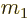and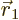be the mass and po­si­tion of the mas­sive body (earth or pro­ton), and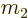and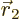those of the lighter one (moon or elec­tron). Clas­si­cally the force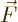be­tween the masses will be a func­tion of the dif­fer­ence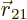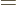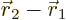in their po­si­tions. In the naive ap­proach the heavy mass is as­sumed to be at rest at the ori­gin. Then, and so the naive equa­tion of mo­tion for the lighter mass is, ac­cord­ing to New­ton’s sec­ond law,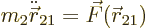Now con­sider the true mo­tion. The cen­ter of grav­ity is de­fined as a mass-weighted av­er­age of the po­si­tions of the two masses: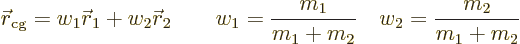It is shown in ba­sic physics that the net ex­ter­nal force on the sys­tem equals the to­tal mass times the ac­cel­er­a­tion of the cen­ter of grav­ity. Since in this case it will be as­sumed that there are no ex­ter­nal forces, the cen­ter of grav­ity moves at a con­stant ve­loc­ity. There­fore, the cen­ter of grav­ity can be taken as the ori­gin of an in­er­tial co­or­di­nate sys­tem. In that co­or­di­nate sys­tem, the po­si­tions of the two masses are given by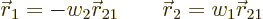be­cause the po­si­tion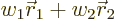of the cen­ter of grav­ity must be zero in this sys­tem, and the dif­fer­encemust be. (Note that the sum of the two weight fac­tors is one.) Solve these two equa­tions forandand you get the re­sult above.

The true equa­tion of mo­tion for the lighter body is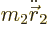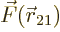, or plug­ging in the above ex­pres­sion forin the cen­ter of grav­ity sys­tem,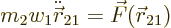That is ex­actly the naive equa­tion of mo­tion if you re­placein it by the re­duced mass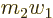, i.e. by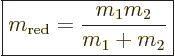(A.16)

The re­duced mass is al­most the same as the lighter mass if the dif­fer­ence be­tween the masses is large, like it is in the cited ex­am­ples, be­cause thencan be ig­nored com­pared toin the de­nom­i­na­tor.

The bot­tom line is that the mo­tion of the two-body sys­tem con­sists of the mo­tion of its cen­ter of grav­ity plus mo­tion around its cen­ter of grav­ity. The mo­tion around the cen­ter of grav­ity can be de­scribed in terms of a sin­gle re­duced mass mov­ing around a fixed cen­ter.

The next ques­tion is if this re­duced mass idea is still valid in quan­tum me­chan­ics. Quan­tum me­chan­ics is in terms of a wave func­tion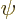that for a two-par­ti­cle sys­tem is a func­tion of bothand. Also, quan­tum me­chan­ics uses the po­ten­tial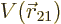in­stead of the force. The Hamil­ton­ian eigen­value prob­lem for the two par­ti­cles is: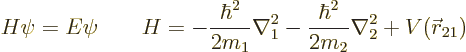where the two ki­netic en­ergy Lapla­cians in the Hamil­ton­ian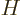are with re­spect to the po­si­tion co­or­di­nates of the two par­ti­cles: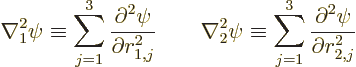Now make a change of vari­ables fromandto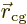andwhere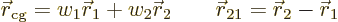The de­riv­a­tives ofcan be con­verted us­ing the chain rule of dif­fer­en­ti­a­tion: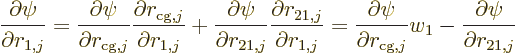or dif­fer­en­ti­at­ing once more and sum­ming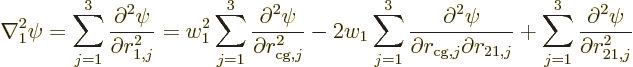and a sim­i­lar ex­pres­sion for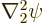, but with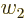in­stead of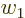and a plus sign in­stead of the mi­nus sign. Com­bin­ing them to­gether in the Hamil­ton­ian, and sub­sti­tut­ing forand, the mixed de­riv­a­tives drop out against each other and what is left is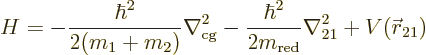The first term is the ki­netic en­ergy that the to­tal mass would have if it was at the cen­ter of grav­ity; the next two terms are ki­netic and po­ten­tial en­ergy around the cen­ter of grav­ity, in terms of the dis­tance be­tween the masses and the re­duced mass.

The Hamil­ton­ian eigen­value prob­lem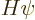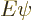has sep­a­ra­tion of vari­ables so­lu­tions of the form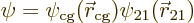Sub­sti­tut­ing this and the Hamil­ton­ian above intoand di­vid­ing by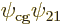pro­duces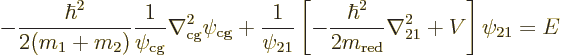Call the first term in the left hand side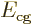and the sec­ond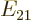. By that de­f­i­n­i­tion,would nor­mally be a func­tion of, be­cause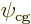is, but since it is equal to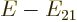and those do not de­pend on,can­not ei­ther, and must be a con­stant. By sim­i­lar rea­son­ing,can­not de­pend onand must be a con­stant too. There­fore, rewrit­ing the de­f­i­n­i­tions ofand, two sep­a­rate eigen­value prob­lems are ob­tained: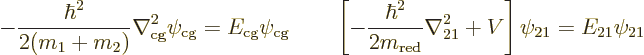The first de­scribes the quan­tum me­chan­ics of an imag­i­nary to­tal mass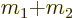lo­cated at the cen­ter of grav­ity. The sec­ond de­scribes an imag­i­nary re­duced mass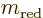at a lo­ca­tionaway from a fixed cen­ter that ex­pe­ri­ences a po­ten­tial.

For the hy­dro­gen atom, it means that if the prob­lem with a sta­tion­ary pro­ton is solved us­ing an re­duced elec­tron mass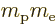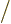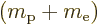, it solves the true prob­lem in which the pro­ton moves a bit too. Like in the clas­si­cal analy­sis, the quan­tum analy­sis shows that in ad­di­tion the atom can move as a unit, with a mo­tion de­scribed in terms of its cen­ter of grav­ity.

It can also be con­cluded, from a slight gen­er­al­iza­tion of the quan­tum analy­sis, that a con­stant ex­ter­nal grav­ity field, like that of the sun on the earth-moon sys­tem, or of the earth on a hy­dro­gen atom, causes the cen­ter of grav­ity to ac­cel­er­ate cor­re­spond­ingly, but does not af­fect the mo­tion around the cen­ter of grav­ity at all. That re­flects a ba­sic tenet of gen­eral rel­a­tiv­ity.